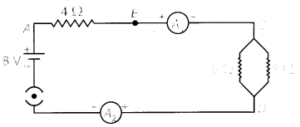# Chapter 12 – Electricity Questions and Answers: NCERT Solutions for Class 10 Science

Class 10 Science NCERT book solutions for Chapter 12 - Electricity Questions and Answers.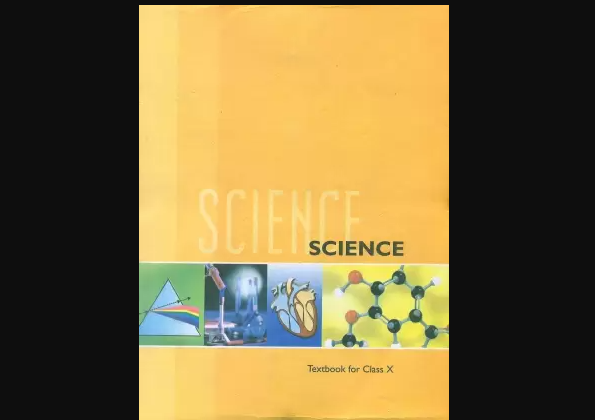##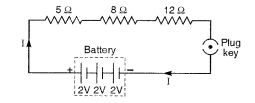Question 2 Redraw the circuit of Questions 1, putting in an ammeter to measure the current through the resistors and a voltmeter to measure the potential difference across the 12 Ω resistor. What would be the readings in the ammeter and the voltmeter ?

### Solution: The required circuit diagram is shown on the right. Total voltage, V = 3 x 2 = 6V Total resistance, R = 5Ω + 8Ω + 12Ω = 25Ω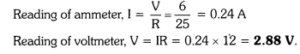## Question 6 A copper wire has diameter 0.5 mm and resistivity of 1.6 x 10-8 Ω m. What will be the length of this wire to make its resistance 10 Ω ? How much does the resistance change if the diameter is doubled ?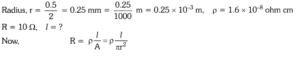##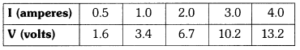Plot a graph between V and I and calculate the resistance of the resistor.

### Solution: The graph between V and I for the above data is given below. The slope of the graph will give the value of resistance. Let us consider two points P and Q on the graph. and from P along Y-axis, which meet at point R. Now, QR = 10.2V – 34V = 6.8V And PR = 3 – 1 = 2 ampere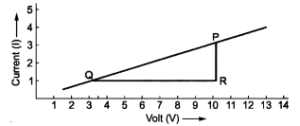## Question 11 Show how you would connect three resistors, each of resistance 6 Ω, so that the combination has a resistance of (i) 9 Ω, (ii) 4Ω

### Solution: Here, R1 = R2 = R3 = 6 Ω. (i) When we connect R1 in series with the parallel combination of R2 and R3 as shown in Fig. (a). The equivalent resistance is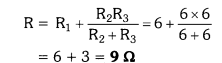## Question 13 A hot plate of an electric oven connected to a 220 V line has two resistance coils A and B, each of 24 Ω resistance, which may be used separately, in series, or in parallel. What are the currents in the three cases ?

### Solution: (i) When the two coils A and B are used separately. R = 24 Ω, V = 220 V### (ii) When the two coils are connected in series,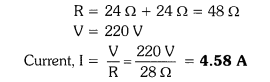## (iii) When the two coils are connected in parallel.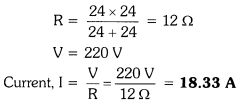Question 14 Compare the power used in the 2 Ω resistor in each of the following circuits (i) a 6 V battery in series with 1 Ω and 2 Ω resistors, and (ii) a 4 V battery in parallel with 12 Ω and 2 Ω resistors.

### Solution: (i) The circuit diagram is shown in figure. Total resistance, R = 1Ω + 2Ω = 3Ω Potential difference, V = 6 V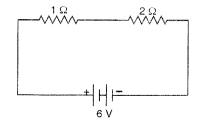##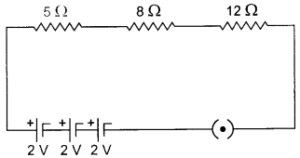Question 2: Redraw the circuit of the above question, putting in an ammeter to measure the current through the resistors and a voltmeter to measure the voltage across the 12 resistor. What would be the reading in the ammeter and the voltmeter?

###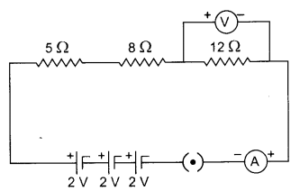Total resistance of the circuit = R Since all the three resistors are connected in series, so, the equivalent resistance R is equal to the sum of all resistance. R = 5 Ω + 8 Ω + 12 Ω = 25 Ω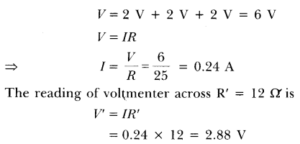## Question 1: A piece of wire of resistance R is cut into five equal parts. These parts are then connected in parallel. If the equivalent resistance of this combination is R’, then the ratio R/R’ is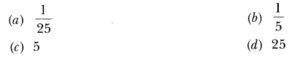## Question 6: A copper wire has a diameter 0.5 mm and resistivity of 1.6 X 10-8 Ωm. What will be the length of this wire to make its resistance 10 Ω? How much does the resistance change if the diameter is doubled?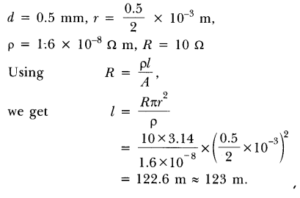##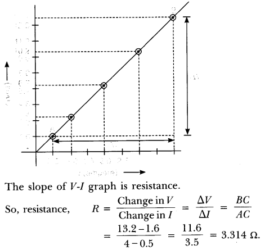Question 8: When a 12 V battery is connected across an unknown resistor, there is a current of 2.5 mA in the circuit. Find the value of the resistance of the resistor.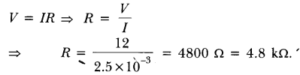##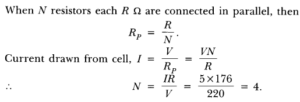Question 11: Show how you would connect three resistors, each of resistance 6 Ω , so that the combination has a resistance of (i) 9 Ω , (it) 4 Ω .

### Answer: (i) When two 6 Ω resistances are in parallel and the third is in combination to this, the equivalent resistance will be 9 Ω.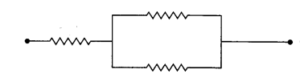## Question 13 A hot plate of an electric oven connected to a 220 V line has two resistance coils A and B, each of 24 Ω resistance, which may be used separately, in series, or in parallel. What are the currents in the three cases ?

### Solution: (i) When the two coils A and B are used separately. R = 24 Ω, V = 220 V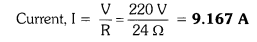##Question 14 Compare the power used in the 2 Ω resistor in each of the following circuits (i) a 6 V battery in series with 1 Ω and 2 Ω resistors, and (ii) a 4 V battery in parallel with 12 Ω and 2 Ω resistors.

### Solution: (i) The circuit diagram is shown in figure. Total resistance, R = 1Ω + 2Ω = 3Ω Potential difference, V = 6 V##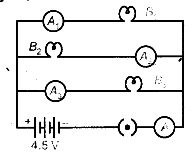(i) What happens to the glow of the other two bulbs when the bulb B j gets fused? (ii) What happens to the reading of A1 ,A2 , A3 and A when the bulb B2 gets fused? (iii) How much power is dissipated in the circuit when all the three bulbs glow together?

### Answer: (i) Since B1 ,B2 and B3 are in parallel, the potential difference across each of them will remain same. So when the bulb B1 gets fused, B2 and B3 have the same potential and continues with the same energy dissipated per second, i.e. they will glow continuously as they were glowing before.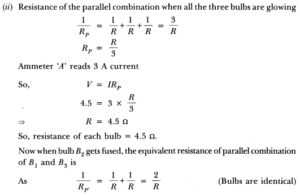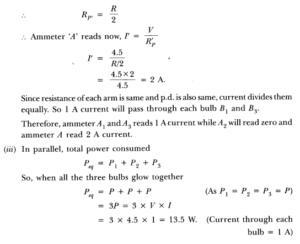## Question 2: Find out the following in the electric circuit given in figure: